Get instant live expert help with Excel or Google Sheets“My Excelchat expert helped me in less than 20 minutes, saving me what would have been 5 hours of work!”

#### Post your problem and you’ll get Expert help in seconds.

Your message must be at least 40 characters
Our professional Expert are available now. Your privacy is guaranteed.

# Excel COUNT Examples – How to Use COUNT, COUNT Greater Than, COUNT if Not Blank

The COUNT function in Excel adds up the number of cells that contain your specified numbers, text, dates, or that meet other criteria. Not only are there many ways to use the COUNT function, but there are also other ways to “count” items in Excel.

This is a list of the Excelchat tutorials that deal with both the COUNT function and “counting” items in an Excel workbook. It’s a long list, so if you want to skip it and get some immediate help, Excelchat’s live chat feature is always standing by to help.Learn how to count values and cells in Excel with these COUNT tutorials in Excelchat.

## How to Count Duplicate Values in Excel

This tutorial explains how you can count duplicate values in Excel. Specifically, you can accomplish this using the COUNTIF function. It provides the formula for the action as well as an example with illustrations for duplicate rows and case-sensitive duplicates.

## How to Count Unique and Distinct Values in Excel

If you need to count unique and distinct values in Excel, you can learn to do this by reading this piece. It describes how to find out how many unique values (no duplicates) are in a specified range by using a combination of the SUM and COUNTIF functions.

## How to Count Colored or Highlighted Cells in Excel

The COUNT function in Excel doesn’t allow you to determine the number of colored or highlighted cells in the selection, but this tutorial provides several different workarounds to accomplish this. There are examples and also a video tutorial to use as a guide.

## Count Cells Not Equal To

When you count cells in Excel, you might want to find the ones that are NOT equal to certain named criteria. This piece explains how to do this using the COUNTIF function. It includes the syntax for the formulas as well as a detailed example.

## How to Count Non-Blank or Non-Empty Cells in Excel

This tutorial shows the reader how to find out the number of cells in a range that are populated, meaning they are not empty or blank. It shows how to do this using the Find function in Excel as well as the COUNTA function – including examples.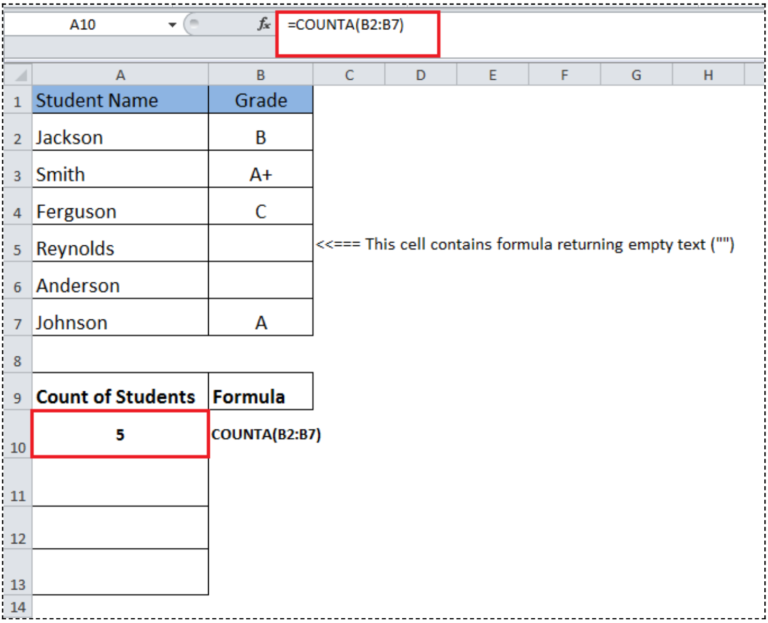## How to Count Cells that Contain Specific Text

When you need to count the number of cells in Excel that contain certain text, this tutorial can teach you how to accomplish this task. It will guide you through using the COUNTIF function to specify your range and criteria, and there is an example with illustrations.

## How to Count Words in Excel

Excel provides several functions that allow you to count cells based on certain criteria. If you need to count words in Excel, this piece shows how to do this using a combination of the SUM, LEN, TRIM, and SUBSTITUTE functions. It includes examples.

## How to Count Unique Text Values in a Range in Excel

Assuming you have a range of cells in Excel and you want to count the unique text values, this guide can help. It shows how to accomplish this in two ways: using the COUNTIF function or a combination of the SUMPRODUCT, FREQUENCY, MATCH, and ROW functions.

## How to Count Cells that Contain Negative Numbers in Excel

Do you need to find out the number of cells in a range that contain negative numbers? This tutorial explains how you can do this using the COUNTIF function to specify your range and criteria. It includes an example and illustrations.

## Learn How to Count Occurrences in an Entire Workbook

In addition to counting within a sheet or range, you can also count the number of occurrences of something within an entire Excel workbook. This piece shows how to do this by first naming the sheets and then combining the SUMPRODUCT, COUNTIF, and INDIRECT functions.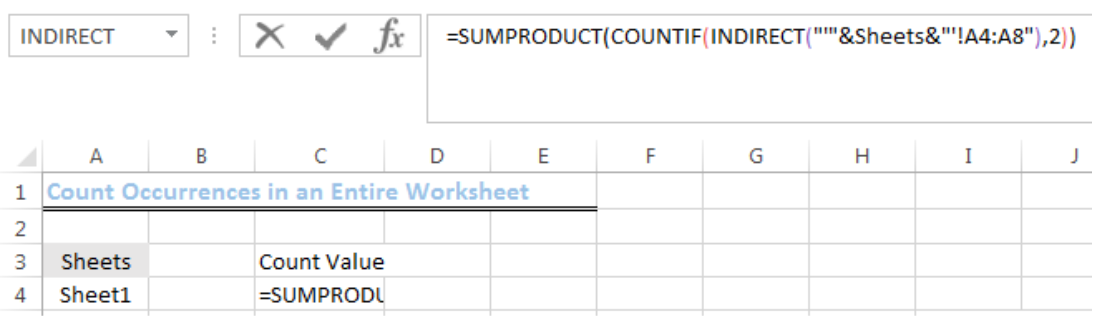## Count Cells that Are Not Blank

This is another tutorial piece that illustrates how to count the number of cells in a sheet that are not blank. It uses the COUNTA function and gives the syntax for the formula before providing an example with illustrations.

## How to Count Cells That Do Not Contain Certain Criteria

If you want to count the cells that don’t contain certain criteria, this piece explains how to do this. Depending on whether you have a single criteria or several, you will use either the COUNTIF or COUNTIFS function. Examples are included.

## Count Visible Rows Only with Criteria

Excel gives you the option to hide rows and columns, and you might only want to count visible rows with certain criteria. This tutorial shows how you can do this by using a combination of the SUMPRODUCT, SUBTOTAL, OFFSET, MIN, and ROW functions.

## Count Rows That Contain Specific Text in Excel

This piece explains how the reader can count the rows in Excel that contain specified text. It uses a combination of the SUM, MMULT, TRANSPOSE, and COLUMN function. There is an explanation of the formula and examples included.

## How to Count Text in Excel

If you need a simple way to count your text in Excel, this tutorial shows you how to do this using the COUNTIF function. It provides the syntax for the formula and then several examples with illustrations.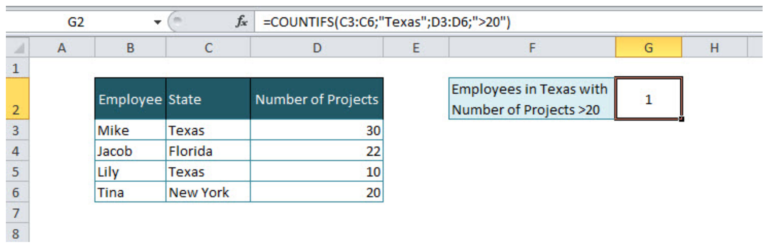## How to Count Cells That Contain Odd Numbers in Excel

Do you only need to count the cells containing odd numbers in Excel? This piece shows the reader how to accomplish this using the SUMPRODUCT and MOD functions. There is not only an example with illustrations, but also a video tutorial included.

## How to Count Dates by Day of the Week in Excel

Counting the number of weekdays days in a date range might be useful, and this tutorial shows how you can do this using a combination of the SUMPRODUCT and WEEKDAY functions. It includes examples and illustrations as a guide.

## How to Count Blank or Empty Cells in Excel

Here is another guide that illustrates how you can count empty or blank cells in Excel. Specifically, it shows how to do this using the program’s built-in “Find” capability as well as the COUNTBLANK function.

## How to Count Unique Values in a Range with COUNTIF

If you want to count values and leave out duplicates, this tutorial shows how to use the COUNTIF function to find unique values in a range in Excel. It combines COUNTIF with the SUMPRODUCT function and includes an example.

## Count Cells Between Dates

This piece shows how you can count the number of cells between two specified dates in Excel. It uses the COUNTIFS function for this operation and provides the reader with an example and illustrations.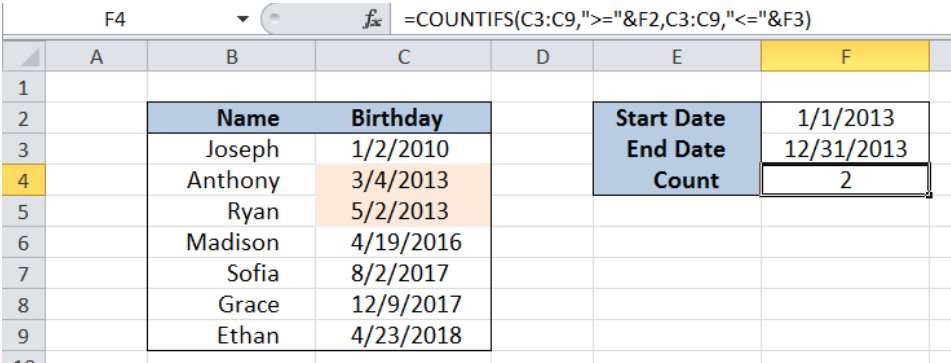## Basic Timesheet Formula with Breaks

This tutorial shows the reader how to create a basic timesheet formula in Excel that includes breaks. You can do this using the MOD function. It provides the syntax for the basic formula and then gives an example with illustrations.

## Count Cells that Contain Numbers

Your Excel spreadsheet might contain cells with numbers, text, and some that are blank. This piece shows how you can count only the cells that contain numbers by using the COUNT function. It includes an example.

## How to Count Characters in Excel

Instead of just counting words or cells, you might also want to count characters in Excel. This piece shows how you can accomplish this by using a combination of the SUMPRODUCT and LEN functions. It includes an example with illustrations.

## Count Cells that Begin With

Do you need to count only the cells that begin with a certain number or character? If so, this tutorial shows you how to do this by using the COUNTIF function. It explains the formula and then gives an example with illustrations.

## Learn How to Count Matches Between Two Columns in Excel

This piece shows the reader how to count the matches between two columns in Excel using the SUMPRODUCT function. It provides the syntax of the formula, shows how to set up the data, and then walks through an example.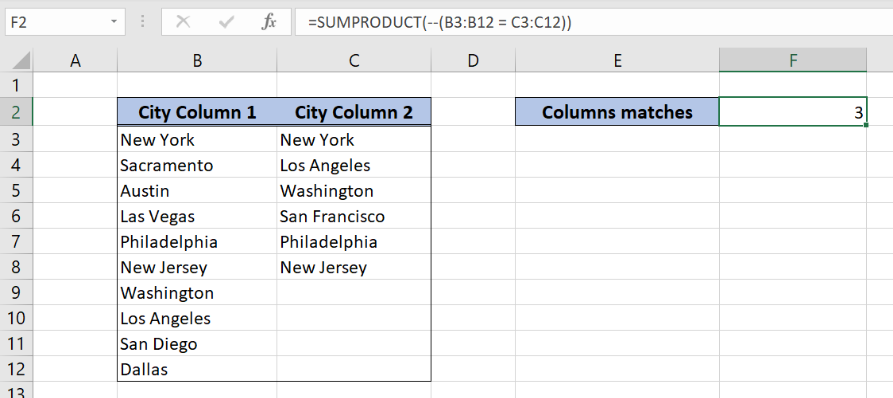## How to Count Unique Numeric Values with Criteria

This tutorial provides the reader with a different way to count the unique numeric values in Excel with criteria. Specifically, it combines the SUM, FREQUENCY, and IF functions to accomplish this goal and provides an example.

## How to Use the COUNT Function in Excel

This is a high-level piece that illustrates how to use the COUNT function in Excel. It explains the purpose of the function, shows the syntax of the formula, and provides various examples of its use to “count” cells in a spreadsheet.

## Using COUNTIF to Find a Running Count of an Occurrence in a List

If you have a large list of data, you might want a cell that keeps a running count of a specific occurrence. This tutorial shows how to accomplish this using the COUNTIF function. It shows how to set up the data and includes an example.

## How to Count Cells Greater Than

This piece serves as a guide on counting the cells in a range that are greater than a specified figure. It uses the COUNTIF function to get this done. You’ll get the syntax for the formula as well as an example with illustrations.

## Count Cells That Are Blank

This is another guide that shows how to count the cells that are blank, or empty, in Excel. In this piece, you’ll use the COUNTBLANK function to accomplish this. This guide includes the formula syntax and a detailed example.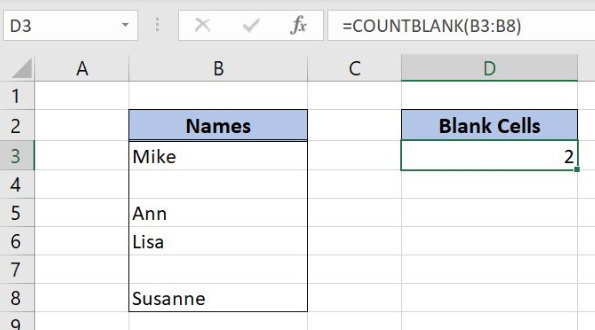## Count If Row Meets Multiple Criteria Internal Criteria

If you need to count the number of rows that meet your multiple criteria, you might need some guidance. This tutorial shows how you can get this done by using the SUMPRODUCT function to set up your conditions. It includes an example.

## Count Cells That Do Not Contain Errors

This piece illustrates how you can count the number of cells that don’t contain errors in Excel. It combines the SUMPRODUCT, NOT, and ISERROR functions. A detailed example with illustrations is included.

## Summary Count with Percentage Breakdown

This step by step guide shows the reader how to create a summary count with a percentage breakdown for a set of specified data. It uses both the COUNTIF and COUNTA functions to accomplish this. There is an example with illustrations.

## Count Cells that Contain Either A or B

When you have either/or criteria for your cell count, this tutorial shows how to do this by combining the SUMPRODUCT, ISNUMBER, and FIND functions. The guide shows how to set up this complex formula and includes a detailed example.

## Count Numbers by Range with COUNTIFS

This piece shows how you can determine how many numbers fall within your specified range. It uses the COUNTIFS function to get this done. There is a detailed example with illustrations included as a guide.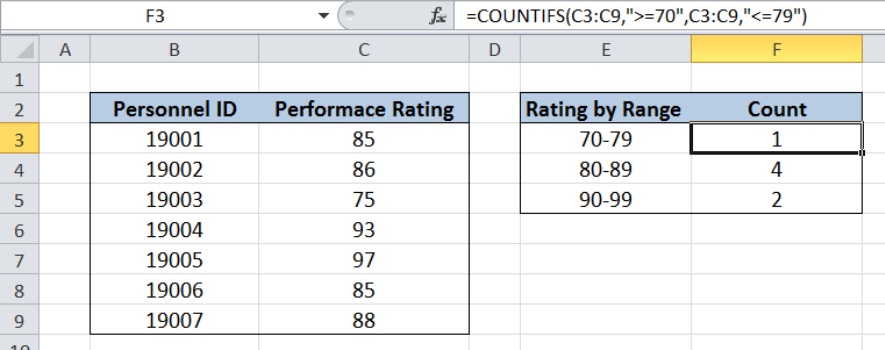## Count Cells Equal to

When your counting criteria is specific, you can count cells equal to using the COUNTIF function. This assumes a single criterion, and this piece shows how to accomplish this will several detailed examples.

## How to Count Cells that Contain Text

Assuming you only want to count cells that contain text, this tutorial will show you how to do this using the COUNTIF function. Specifically, it shows how to use the wildcard (“*”) with COUNTIF in an example as well as how to find cells without text.

## Count Cells Between Two Numbers

This piece shows the reader how to count the number of cells between two numbers using the COUNTIFS function. It gives the syntax for the formula, shows how to set up the data, and then provides an example with illustrations.

## Count Items in a List

This piece explains how to use the COUNTIFS function to count the items in a specified list. It gives the reader several examples as a guide.

This was an exhaustive list, and you likely found what you needed here. If you didn’t, or would just like some more personalized help, Excelchat can deliver. We have Excel experts standing by to help you learn Excel or troubleshoot a problem-specific issue. Your first session is always free.